# 基于Opencv的数字图像处理技巧（python）

## opencv图像处理基础实战

OpenCV是一个基于BSD许可（开源）发行的跨平台计算机视觉机器学习软件库，可以运行在LinuxWindowsAndroidMac OS操作系统上。 它轻量级而且高效——由一系列 C 函数和少量 C++ 类构成，同时提供了Python、Ruby、MATLAB等语言的接口，实现了图像处理和计算机视觉方面的很多通用算法。OpenCV用C++语言编写，它具有C ++，PythonJavaMATLAB接口，并支持Windows，Linux，Android和Mac OS，OpenCV主要倾向于实时视觉应用，并在可用时利用MMX和SSE指令， 如今也提供对于C#、Ch、Ruby，GO的支持。下面的工作是基于Python进行编码的。

import cv2
from matplotlib import pyplot as plt
import numpy as np图1、高速公路图原图

img_path = "C:\\Users\\Lijian\\Desktop\\timg.jpg"
img.shape

运行上段代码的结果：

(511, 860, 3)

gray_image = cv2.cvtColor(img, cv2.COLOR_BGR2GRAY)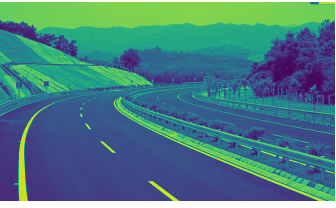gray_image = cv2.cvtColor(img, cv2.COLOR_BGR2GRAY)
plt.imshow(gray_image,cmap="gray")
plt.axis('off')
print(gray_image.shape)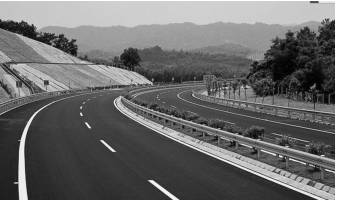如图3所示，我们已将图像转换为黑白图像。当我们检查其尺寸时，不再有3个尺寸，仅为(511, 860)的矩阵。
(thresh, blackAndWhiteImage) = cv2.threshold(gray_image, 200, 255, cv2.THRESH_BINARY)
(thresh, blackAndWhiteImage) = cv2.threshold(gray_image, 80, 255, cv2.THRESH_BINARY)
(thresh, blackAndWhiteImage) = cv2.threshold(gray_image, 160, 255, cv2.THRESH_BINARY)
(thresh, blackAndWhiteImage) = cv2.threshold(gray_image, 200, 255, cv2.THRESH_BINARY)
plt.imshow(blackAndWhiteImage,cmap="gray")
plt.axis('off')
plt.show()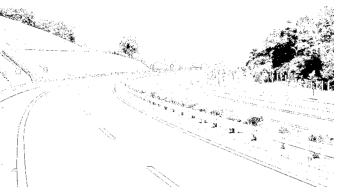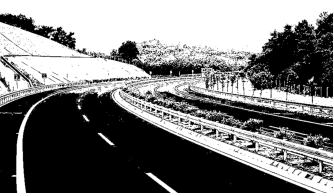a（20，255）                                                                       b（80，255）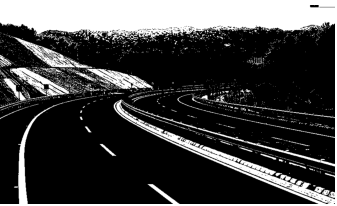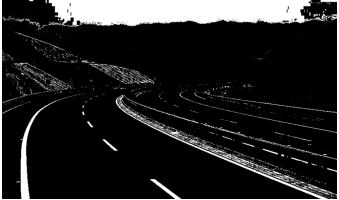c（160，255）                                                                    d（200，255）

OpenCV中阈值功能所需的第一个参数是要处理的图像。以下参数是阈值。第三个参数是我们要分配超出阈值的矩阵元素的值。可以在图3中看到四个不同阈值的影响。在第一张图像（图像1）中，该阈值确定为20.将20之上的所有值分配给255.其余值为设置为0。这仅允许黑色或非常深的颜色为黑色，而所有其他阴影直接为白色。图像2和图像3的阈值分别为80和160.最后，在图像4中将阈值确定为200.与图像1不同，白色和非常浅的颜色被指定为255，而所有在图4中将剩余值设置为0。

output2 = cv2.blur(gray_image, (10, 10))
plt.imshow(output2,cmap="gray")
plt.axis('off')
plt.show()

output2 = cv2.GaussianBlur(gray_image, (9, 9), 5)
plt.imshow(output2,cmap="gray")
plt.axis('off')
plt.show()a均值滤波                                                                 b高斯滤波

## 导向滤波代码：

import numpy as np
import cv2

def guideFilter(I, p, winSize, eps):

mean_I = cv2.blur(I, winSize)      # I的均值平滑
mean_p = cv2.blur(p, winSize)      # p的均值平滑

mean_II = cv2.blur(I * I, winSize) # I*I的均值平滑
mean_Ip = cv2.blur(I * p, winSize) # I*p的均值平滑

var_I = mean_II - mean_I * mean_I  # 方差
cov_Ip = mean_Ip - mean_I * mean_p # 协方差

a = cov_Ip / (var_I + eps)         # 相关因子a
b = mean_p - a * mean_I            # 相关因子b

mean_a = cv2.blur(a, winSize)      # 对a进行均值平滑
mean_b = cv2.blur(b, winSize)      # 对b进行均值平滑

q = mean_a * I + mean_b
return q

if __name__ == '__main__':
eps = 0.01
winSize = (5,5)
image = cv2.resize(image, None,fx=0.7, fy=0.7, interpolation=cv2.INTER_CUBIC)
I = image/255.0        #将图像归一化
p =I
guideFilter_img = guideFilter(I, p, winSize, eps)

# 保存导向滤波结果
guideFilter_img  = guideFilter_img  * 255
guideFilter_img [guideFilter_img  > 255] = 255
guideFilter_img  = np.round(guideFilter_img )
guideFilter_img  = guideFilter_img.astype(np.uint8)
cv2.imshow("image",image)
cv2.imshow("winSize_5", guideFilter_img )
cv2.waitKey(0)
cv2.destroyAllWindows()(h, w) = img.shape[:2]
center = (w / 2, h / 2)
M = cv2.getRotationMatrix2D(center, 13, scale  =1.1)
rotated = cv2.warpAffine(gray_image, M, (w, h))
plt.imshow(rotated,cmap="gray")
plt.axis('off')
plt.show()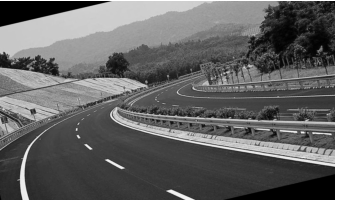## 项目一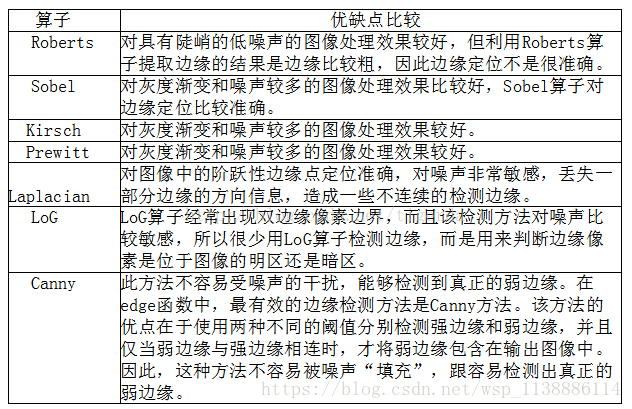canny算子

(h, w) = img.shape[:2]
center = (w / 2, h / 2)
M = cv2.getRotationMatrix2D(center, 13, scale  =1.1)
rotated = cv2.warpAffine(gray_image, M, (w, h))
plt.imshow(rotated,cmap="gray")
plt.axis('off')
plt.show()

sobel算子

img = cv2.imread(img_path)

gray_image = cv2.cvtColor(img, cv2.COLOR_BGR2GRAY)

(thresh, output2) = cv2.threshold(gray_image, 200, 255, cv2.THRESH_BINARY)

output2 = cv2.GaussianBlur(output2, (5, 5), 3)

output2 = cv2.Sobel(output2,cv2.CV_16S,1,0)

plt.imshow(output2,cmap="gray")
plt.axis('off')

plt.show()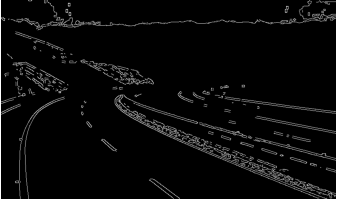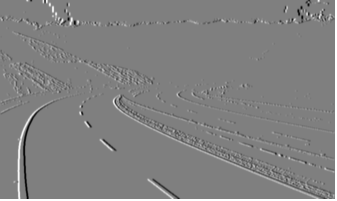a.canny算子                                                                      b.sobel算子

Canny边缘检测算子是一种多级检测算法。1986年由John F. Canny提出，同时提出了边缘检测的三大准则：

• 低错误率的边缘检测：检测算法应该精确地找到图像中的尽可能多的边缘，尽可能的减少漏检和误检。
• 最优定位：检测的边缘点应该精确地定位于边缘的中心。
• 图像中的任意边缘应该只被标记一次，同时图像噪声不应产生伪边缘。

Canny算法出现以后一直是作为一种标准的边缘检测算法，此后也出现了各种基于Canny算法的改进算法。时至今日，Canny算法及其各种变种依旧是一种优秀的边缘检测算法。而且除非前提条件很适合，你很难找到一种边缘检测算子能显著地比Canny算子做的更好。

1. 高斯模糊。2. 计算梯度幅值和方向。3. 非最大值抑制。4. 双阀值。5. 滞后边界跟踪。

canny算子详解

canny算子调整参数进一步细化

img = cv2.imread(img_path)
gray_image = cv2.cvtColor(img, cv2.COLOR_BGR2GRAY)
(thresh, output2) = cv2.threshold(gray_image, 245, 255, cv2.THRESH_BINARY)
output2 = cv2.Canny(output2, 180, 255)
plt.imshow(output2,cmap="gray")
plt.axis('off')
plt.show()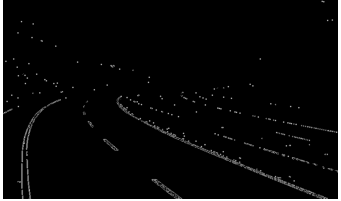lines = cv2.HoughLinesP(output2, 1, np.pi/180,30)
for line in lines:
x1,y1,x2,y2 = line
cv2.line(img,(x1,y1),(x2,y2),(0,255,0),4)
cv2.imshow("image",img)
cv2.waitKey(0)
cv2.destroyAllWindows()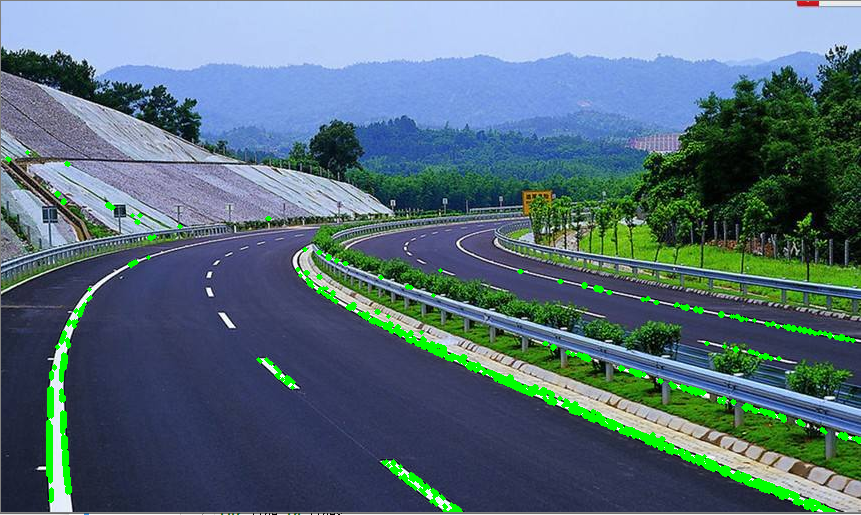def mask_of_image(image):
height = image.shape
polygons = np.array([[(0,height),(2200,height),(250,100)]])
return masked_image

out=mask_of_image(output2)
cv2.imshow("image",out)
cv2.waitKey(0)
cv2.destroyAllWindows()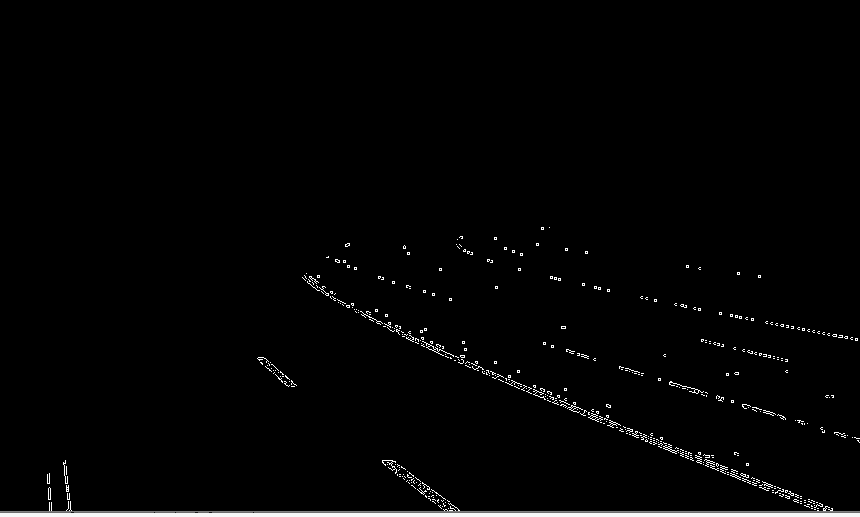lines = cv2.HoughLinesP(out, 1, np.pi/180,30)
for line in lines:
x1,y1,x2,y2 = line
cv2.line(img,(x1,y1),(x2,y2),(0,255,0),4)
cv2.imshow("image",img)
cv2.waitKey(0)
cv2.destroyAllWindows()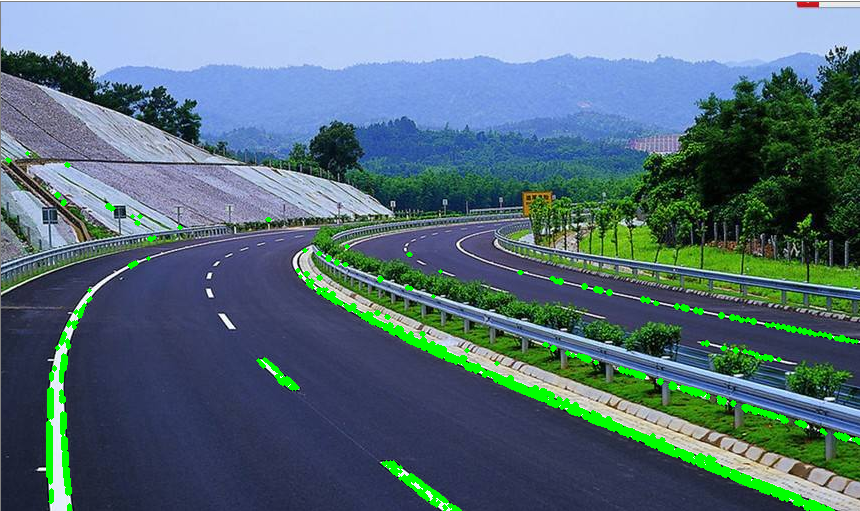09-17725706-14639
05-28272
02-033401
05-158185
10-291976
06-09
12-084701
05-28237